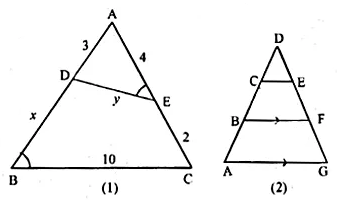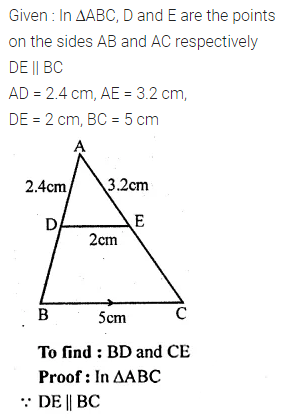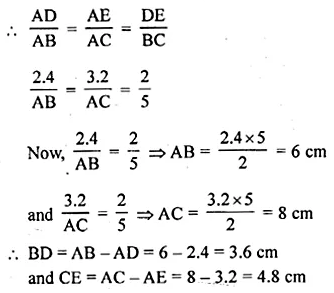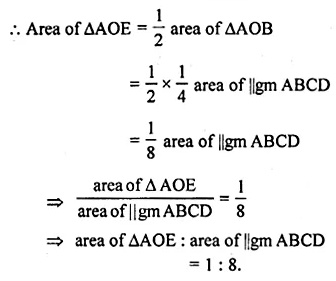ML Aggarwal Class 10 Solutions for ICSE Maths Chapter 13 Similarity Chapter Test

ML Aggarwal Class 10 Solutions for ICSE Maths Chapter 13 Similarity Chapter Test

ML Aggarwal Class 10 Solutions for ICSE Maths Chapter 13 Similarity Chapter Test

Question 1.
In the given figure, ∠1 = ∠2 and ∠3 = ∠4. Show that PT × QR = PR × ST.
Solution:Question 2.
In the adjoining figure, AB = AC. If PM ⊥ AB and PN ⊥ AC, show that PM × PC = PN × PB.Solution:Question 3.
(a) In the figure (1) given below. ∠AED = ∠ABC. Find the values of x and y.
(b) In the fig. (2) given below, CD = $$\\ \frac { 1 }{ 2 }$$ AC, B is mid-point of AC and E is mid-point of DF. If BF || AG, prove that:
(i) CE || AG
(ii) 3ED = GD.Solution:Question 4.
In the given figure, 2AD = BD, E is mid-point of BD and F is mid-point of AC and EC || BH. Prove that:
(i) DF || BH
(ii) AH = 3AF.Solution:Question 5.
In a ∆ABC, D and E are points on the sides AB and AC respectively such that DE || BC. If AD = 2.4 cm, AE = 3.2 cm, DE = 2 cm and BC = 5 cm, find BD and CE.
Solution:Question 6.
In a ∆ABC, D and E are points on the sides AB and AC respectively such that AD = 5.7 cm, BD = 9.5 cm, AE = 3.3 cm and AC = 8.8cm. Is DE || BC? Justify your answer.
Solution:Question 7.
In a ∆ABC, DE is parallel to the base BC, with D on AB and E on AC. If $$\frac { AD }{ DB } =\frac { 2 }{ 3 } ,\frac { BC }{ DE }$$
Solution:Question 8.
If the area of two similar triangles are 360 cm² and 250 cm² and if one side of the first triangle is 8 cm, find the length of the corresponding side of the second triangle.
Solution:Question 9.
In the adjoining figure, D is a point on BC such that ∠ABD = ∠CAD. If AB = 5 cm, AC = 3 cm and AD = 4 cm, find
(i) BC
(ii) DC
(iii) area of ∆ACD : area of ∆BCA.Solution:Question 10.
In the adjoining figure, the diagonals of a parallelogram intersect at O. OE is drawn parallel to CB to meet AB at E, find the area of DAOE : area of ||gm ABCD.Solution:Question 11.
In the given figure, ABCD is a trapezium in which AB || DC. If 2AB = 3DC, find the ratio of the areas of ∆AOB and ∆COD.Solution:Question 12.
In the adjoining figure, ABCD is a parallelogram. E is mid-point of BC. DE meets the diagonal AC at O and meets AB (produced) at F. Prove that(i) DO : OE = 2 : 1
(ii) area of ∆OEC : area of ∆OAD = 1 : 4.
Solution:Question 13.
A model of a ship is made to a scale of 1 : 250. Calculate :
(i) the length of the ship, if the length of the model is 1.6 m.
(ii) the area of the deck of the ship, if the area of the deck of the model is 2.4 m².
(iii) the volume of the model, if the volume of the ship is 1 km³.
Solution: